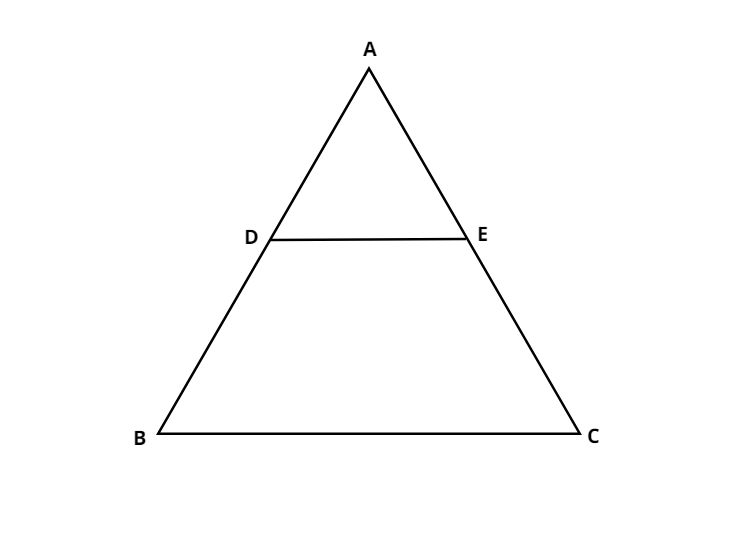Courses
Courses for Kids
Free study material
Offline Centres
MoreLast updated date: 30th Nov 2023
Total views: 381.6k
Views today: 7.81k

# D and E are points on the sides AB and AC respectively such that BD = CE . If $\angle B = \angle C$ , show that $DE\parallel BC$ .Verified
381.6k+ views
Hint: Use properties of triangles to find the equal sides and using the provided information break them to show that line BC is parallel to DE .At first we draw a triangle ABC with point D and point E on sides AB and AC respectively .
Since $\angle B = \angle C$
AB = AC ( sides opposite to equal angles of a triangle are equal )
Now we know that AB = AD + DB
and AC = AE + EC
AD + DB = AE + EC ( from above )
Cancelling out BD and EC as they are equal given in the question , we get
$\dfrac{{AD}}{{BD}} = \dfrac{{AE}}{{CE}}$
Thus, we get $DE\parallel BC$.